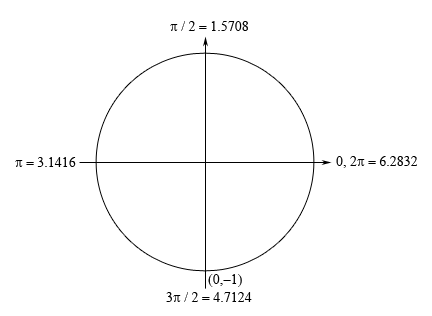Paul's Online Notes
Home / Calculus I / Review / Trig Equations with Calculators, Part I
Show Mobile Notice Show All Notes Hide All Notes
Mobile Notice
You appear to be on a device with a "narrow" screen width (i.e. you are probably on a mobile phone). Due to the nature of the mathematics on this site it is best views in landscape mode. If your device is not in landscape mode many of the equations will run off the side of your device (should be able to scroll to see them) and some of the menu items will be cut off due to the narrow screen width.

### Section 1.5 : Solving Trig Equations with Calculators, Part I

8. Find the solution(s) to $$12\sin \left( {7y} \right) + 11 = 3 + 4\sin \left( {7y} \right)$$ that are in $$\left[ { - 2, - \frac{1}{2}} \right]$$. Use at least 4 decimal places in your work.

Show All Steps Hide All Steps

Hint : Find all the solutions to the equation without regard to the given interval. The first step in this process is to isolate the sine (with a coefficient of one) on one side of the equation.
Start Solution

Isolating the sine (with a coefficient of one) on one side of the equation gives,

$\sin \left( {7y} \right) = - 1$
Hint : Use your knowledge of the unit circle to determine all the angles in the range $$\left[ {0,2\pi } \right]$$ for which sine will have this value.
Show Step 2

If you need to use a calculator to get the solution for this that is fine, but this is also one of the standard angles as we can see from the unit circle below.Because we’re dealing with sine in this problem and we know that the $$y$$-axis represents sine on a unit circle we’re looking for angle(s) that will have a $$y$$ coordinate of –1. The only angle that will have this $$y$$ coordinate will be $$\frac{{3\pi }}{2} = 4.7124$$.

Note that unlike all the other problems that we’ve worked to this point this will be the only angle. There is simply not another angle in the range $$\left[ {0,2\pi } \right]$$ for which sine will have this value. Don’t get so locked into the usual case where we get two possible angles in the $$\left[ {0,2\pi } \right]$$ that when these single solution cases roll around you decide you must have done something wrong. They happen on occasion and we need to be able to deal with them when they occur.

Hint : Using the angle above write down all the angles for which sine will have this value and use these to write down all the solutions to the equation.
Show Step 3

From the discussion in the notes for this section we know that once we have the angle above we can get all possible angles by simply adding “$$+ \,2\pi n$$ for $$n = 0, \pm 1, \pm 2, \ldots$$” onto the angle.

This then means that we must have,

$7y = 4.7124 + 2\pi n\hspace{0.25in}n = 0, \pm 1, \pm 2, \ldots$

Finally, to get all the solutions to the equation all we need to do is divide both sides by 7 and we’ll convert everything to decimals to help with the final step.

\begin{align*}y & = 0.6732 + \frac{{2\pi n}}{7} & \hspace{0.25in} & n = 0, \pm 1, \pm 2, \ldots \\ & = 0.6732 + 0.8976n & \hspace{0.25in} & n = 0, \pm 1, \pm 2, \ldots \end{align*}
Hint : Now all we need to do is plug in values of $$n$$ to determine which solutions will actually fall in the given interval.
Show Step 4

Now let’s find all the solutions. First notice that, in this case, if we plug in positive values of $$n$$ or $$n = 0$$ we will get positive solutions and these will not be in the interval and so there is no reason to even try these. So, let’s start at $$n = - 1$$ and see what we get.

$\begin{array}{lclcl}{n = - 1:\,}&{\require{cancel} \hspace{0.70in} \xcancel{{y = - 0.2244}} > - 0.5}\\{n = - 2:}&{y = - 1.122}\\{n = - 3:}&{\hspace{0.70in} \xcancel{{y = - 2.0196}} < - 2}\end{array}$

So, it looks like we have only a single solution to this equation in the given interval.

$\require{bbox} \bbox[2pt,border:1px solid black]{{y = - 1.122}}$

Note that depending upon the amount of decimals you used here your answers may vary slightly from these due to round off error. Any differences should be slight and only appear around the 4th decimal place or so however.